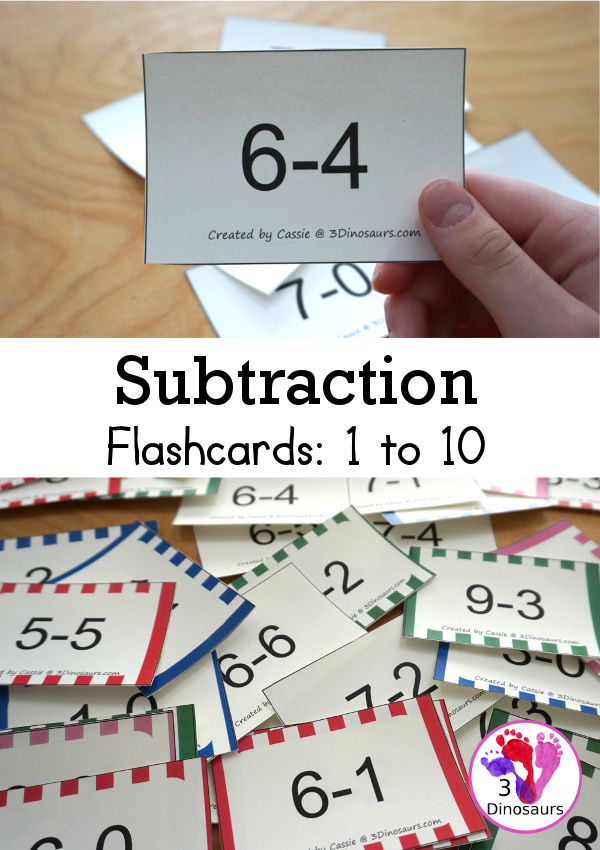# Free Subtraction Flashcards: 1 to 10

These free subtraction flashcards are to help to learn subtraction facts easily. I know that I am always had a time finding just the math flashcards I wanted or they did not have all the options. So I made this set of subtraction cards!

You have numbers subtraction from 1 to 10 in the set. They are easy to print and use the subtraction cards.

I know people have loved the different color options for the math flashcards and this set has the same five color options.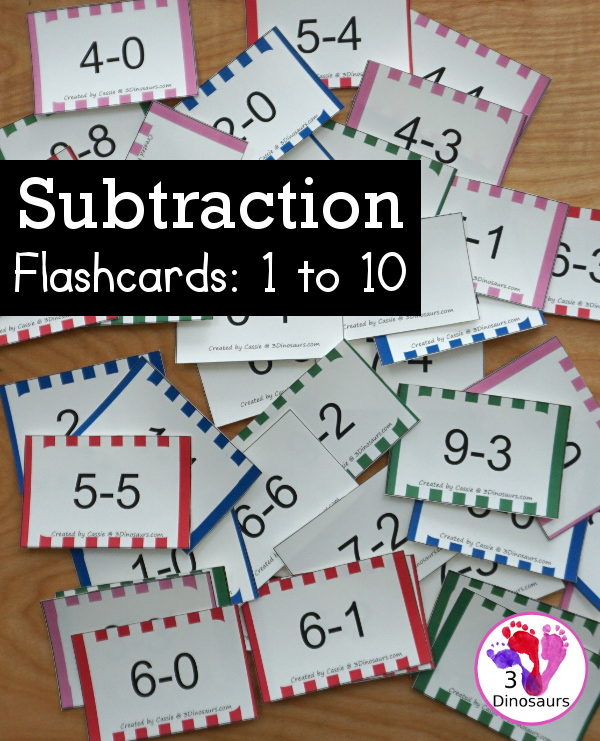We have included links to some of the products and resources we have used with this activity. If you purchase via the links we may earn a small commission.

This is a great addition to the subtraction printables on the site.

## What is Subtraction?

Subtraction is the removal of objects from a collection. So you start with three objects and take one of the objects away and you have two remaining.

The equation for subtraction would look like 3 – 1 = 2.

The subtraction flashcards have 8 subtraction cards on one page. They are in order from 1 to 10. They show all the ways to do subtraction from that number.

There are 5 color options for the printable subtraction flashcards. You have white, red, blue, green, and pink. Each of the colors is a fun way to use a color they like.

These subtraction flashcards work great for kindergarten and first grade.## Things to use with the Subtraction Flashcard Printables

I always get asked what items I use with the subtraction card printables. So here is a list to help you out.

• printer• white cardstock• color cardstock• laminator• laminating pouches• unifix cubes• glass gems• 1 inch ring• mini erasers

## Ways to Use the Subtraction Flashcards Printable

You can work on memorizing subtraction math facts with flashcards, but you don’t have to do just that. You can change up how you use the cards.

### Store Subtraction Flashcards on a Ring

One way you can use the white version of the subtraction flashcards is to print it on color cardstock. Then you can use any mix of colors you want. You can also see that I put them on a ring. The ring helps keep them together. We reuse a lot of our rings. You can also flip through the subtraction flashcards on a ring to review their subtraction facts.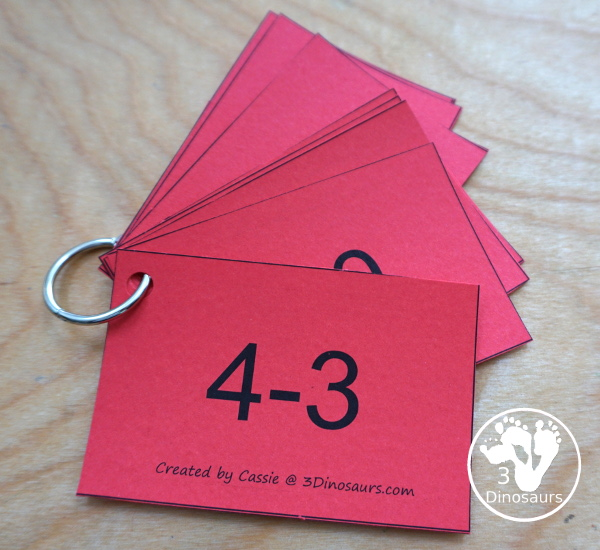### Subtraction Flaschards with Unfix Cubes

You can pull out unifix cubes. I like to match the cube color the color of the subtraction flashcards to make it fun. They can count the total at the start then remove the number they are subtracting to make the answer.### Subtraction Flaschards with Mini Erasers

You can also use mini eraser with the subtraction cards. I use these a lot. I grab ours at the Target dollar section. Well worth it because I can change it up for each season! You could also use glass gemsand change those up during the season.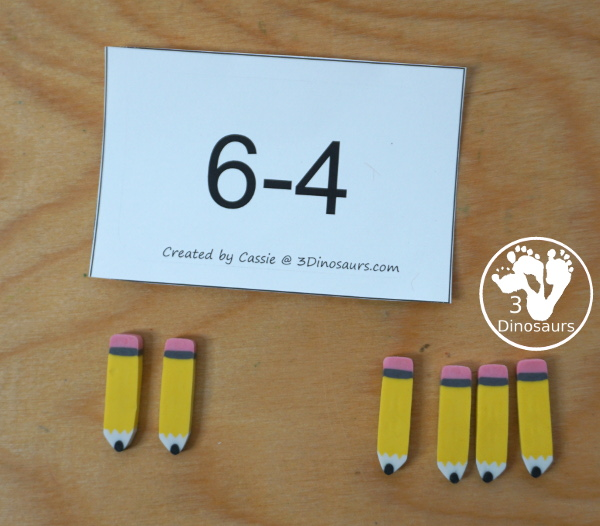### Subtraction Flashcards with Number Bond Mat

These cards are also great with the help mats. I have one of the subtraction number bond mats below. You can see how we used the card to fill out the help mat.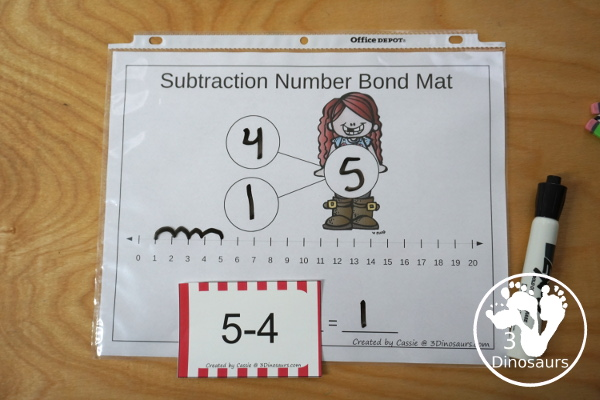You never have to use a flashcard just one way! Keep that in mind when you use them!

## What you will find in the free Subtraction Flashcards 1 to 10 Printables:

• Subtraction from 1 to 10
• 5 flashcard color options: white, red, pink, green and blue
• 10 pages for each color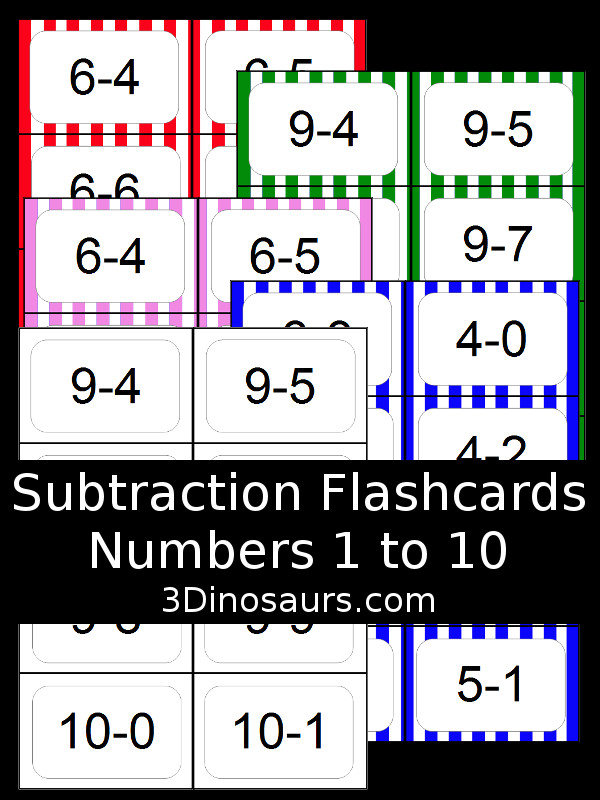## You can check out these other subtraction printables

Hands-On Number Bond Mats: Subtraction

Addition & Subtraction Number Bond Mats with 100 Chart

Math Number Bond Mats Subtraction

Crayon Themed Subtraction Sorting

Subtraction Flash Cards 11 to 20 – You can add these flashcards to the 1 to 10.

Math Fact House: Addition & Subtraction

Check out all the fun SubtractionPrintables & Activities on 3 Dinosaurs.

Cassie – 3Dinosaurs.com